# Fundamentals of Physics + velocity - math problems

#### Number of problems found: 114

• Acceleration 2if a car traveling at a velocity of 80 m/s/south accelerated to a velocity of 100 m/s east in 5 seconds, what is the cars acceleration? using Pythagorean theorem
• Motor physics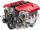Car traveling at 130 km/h on the highway. Pulling power of the motor is 8 kN. Determine engine power in kW.
• Circular motionMass point moves moves uniformly in a circle with radius r = 3.4 m angular velocity ω = 3.6 rad/s. Calculate the period, frequency, and the centripetal acceleration of this movement.
• Ball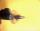The Ball was fired at an angle of 35° at an initial velocity of 292 m/s. Determine the length of the litter. (g = 9.81 m/s2).
• Positional energyWhat velocity in km/h must a body weighing 60 kg have for its kinetic energy to be the same as its positional energy at the height 50 m?
• Free fall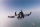How long does the stone fall freely into a depth of 80m? What speed will it hit the bottom of the abyss?
• Free fallThe free fall body has gone 10m in the last 0.5s. Find the body speed at the moment of impact.
• Earth rotation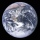How fast is the place on the Earth's equator moving if the Earth's radius is 6378 km?
• Wave parameters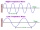Calculate the speed of a wave if the frequency is 336 Hz and the wavelength is 10 m.
• RPMAn electric motor makes 3,000 revolutions per minutes. How many degrees does it rotate in one second?
• A particle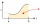A particle moves in a straight line so that its velocity (m/s) at time t seconds is given by v(t) = 3t2-4t-4, t>0. Initially the particle is 8 meters to the right of a fixed origin. After how many seconds is the particle at the origin?
• Time clockWhat is the angle of the hour hand and the minute hand, if it is 0:40?
• The run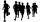On the school playground, the student ran a 60 m track in 9.5 s. What is the average speed of his run?
• Constant Angular Acceleration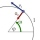The particle began to move from rest along a circle with a constant angular acceleration. After five cycles (n = 5), its angular velocity reached the value ω = 12 rad/s. Calculate the magnitude of the angular acceleration ε of this motion and the time int
• AccelerationThe car accelerates at rate 0.5m/s2. How long travels 400 meters and what will be its speed?
• FlywheelFlywheel turns 450 rev/min (RPM). Determine the magnitude of the normal acceleration of the flywheel point which are at a distance of 10 cm from the rotation axis.
• Train 2The train slowed down from 90 km/h to 72 km/h in 5 seconds. How long track travel?
• Car loop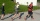The toy car runs at an average speed of 2 m/s. In a minute, it will pass the entire circuit five times. How long is the loop?
• Shot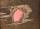Shot with a mass 43 g flying at 256 m/s penetrates into the wood to a depth 25 cm. What is the average force of resistance of wood?
• Walking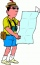Lucie can walk about 3 4/5 miles each hour. How far can she walk in 2 hours 45 minutes?

Do you have an interesting mathematical word problem that you can't solve it? Submit a math problem, and we can try to solve it.

We will send a solution to your e-mail address. Solved examples are also published here. Please enter the e-mail correctly and check whether you don't have a full mailbox.

Please do not submit problems from current active competitions such as Mathematical Olympiad, correspondence seminars etc...

Do you want to convert velocity (speed) units?